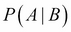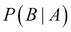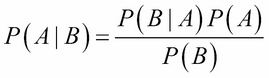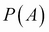•#### Learning Bayesian Models with R#### Overview of this book

Learning Bayesian Models with RCreditswww.PacktPub.comPrefaceFree Chapter
Introducing the Probability TheoryThe R EnvironmentIntroducing Bayesian InferenceMachine Learning Using Bayesian InferenceBayesian Regression ModelsBayesian Classification ModelsBayesian Models for Unsupervised LearningBayesian Neural NetworksBayesian Modeling at Big Data ScaleIndex## Bayesian theorem

From the definition of the conditional probabilitiesand, it is easy to show the following:Rev. Thomas Bayes (1701–1761) used this rule and formulated his famous Bayes theorem that can be interpreted ifrepresents the initial degree of belief (or prior probability) in the value of a random variable A before observing B; then, its posterior probability or degree of belief after accounted for B will get updated according to the preceding equation. So, the Bayesian inference essentially corresponds to updating beliefs about an uncertain system after having made some observations about it. In the sense, this is also how we human beings learn about the world. For example, before we visit a new city, we will have certain prior knowledge about the place after reading from books or on the Web.

However, soon after we reach the place, this belief will get updated based on our initial experience of the place. We continuously update the belief as we explore the new city more and more. We will describe Bayesian inference more in detail in Chapter 3, Introducing Bayesian Inference.